Evolver

CS229 Final Project

Brown University

December 13, 2000

# Abstract

This project produces interesting 2D animations by collaboration between a human and a computer.  The computer assumes the creative role and the human the critical one.  The computer produces multiple images and the user selects the ones he or she likes best.  The computer then produces new images inspired by the ones preferred by the user.  When the user is satisfied, the images become frames of a multi-frame animation sequence.

This work follows the genetic image creation performed by Karl Sims on a CM2, as described in Sims Karl, "Artificial Evolution for Computer Graphics" Computer Graphics, 25(4), July 1991, pp. 319-328.  Sims contributes the general approach of genetic algorithms for evolving interesting image/animation behaviors and his implementation leverages the massive multi-processor architecture of the CM2 via a pixel-based imaging language.

New contributions of this paper are an open-source, platform independent Java implementation that scales from uni-processor to multi-processor machines, an image-based scale-invariant imaging language, breeding of animation behaviors for frames in addition to single images, and presentation of additional implementation details important for success at this approach.

# I.          Introduction

The goal of the project is to produce aesthetically interesting 2D animations by collaboration between a “computer artist,” implemented as genetic algorithm, and a human user.  Individual frames of the animations are encoded in a simple yet powerful language for image filtering called EL (Evolver Language) that was designed for this project.  An infrastructure is provided for combining EL expressions using genetic mating algorithms, as well as a GUI for allowing the human user to interact with the genetic algorithm.  Animations are constructed by interpolating between the expressions that create images for individual frames using a special genetic morph operation.

This is interesting for two reasons. This first is that the human performs a purely critical role in the artistic process by expressing his or her opinion of intermediate works. The 'innovation' is left to the computer. The human user does not have to have any particular artistic skill or knowledge of image processing. It is possible for the user to optionally adjust a number of parameters controlling the genetic algorithms and indirectly affect the creation process as well.

The second reason it is interesting is the staggering complexity and variation of output images given the apparent simplicity of the EL programs generated. It is very difficult for a human to design compact expressions that produce aesthetically pleasing results.  As Sims identifies out in his paper, complicated textures and animations can be procedurally generated.

# II.        The Evolver Language

The EL grammar is given by programming language set notation:

 `  ELValue := ELMatrix | ELScalar | ELVector``  ELMorphInterpolator := ELExpression x ELExpression``  ELOperator := Add | Sub | ...``  ELCall := ELOperator x ELExpression*``  ELExpression := ELCall | ELScalar | ELVector | ELMorphInterpolator`` ``  ELMatrix := ELVector*``  ELVector := ELScalar x ELScalar x ELScalar``  ELScalar := -1 <= s e Reals <= 1`

Figure 1: EL Grammar

The syntax of EL is designed to mimic Java, where functions are applied using the () operator to multiple comma separated arguments. A typical expression looks like:

`Add(Noise(), Blur(Sub (HGradient(), VGradient())))`

Figure 2: Sample EL Expression

For simplicity, all operations are strictly functional, and a behavior (program) is a single expression. All numbers are reals on the range [-1, 1], following Sims's setup. Colors are rendered so that negative values are clamped black. This tends towards black in the images and prevents images from getting too “busy.”

Sims describes an imaging operation as a single function to be evaluated at all pixels. This formulation is natural for the Connection Machine he used for the experiments because it had an array of processors that could each represent one pixel and operate in parallel.

I choose to formulate an imaging operation as a function mapping zero or more images (and some parameters) to an output image.  This allows a more natural inclusion of non-local filters like computing the gradient, edge filters, and blurs.  Sims’s machine used one processor per pixel, so his algorithms were accelerated by pixel independence in the filters.  I used a modern dual-processor PC for my experiments, so losing pixel independence does not affect performance.  This also makes the language simpler because it doesn’t contain context sensitive variables.  Instead of variables like X and Y appearing, I use functions over the whole image such as HGradient().

The following is a list of operators available in EL. The number in parentheses is the number of arguments each takes. Functions of n arguments require at least 2 arguments.

` `
`3D`

These create 3D texture effects.

`EnvironmentMap(2) - Computes surfaces normals as if the second image `
`                      were a height field, then environment map using the`
`                      first.  Creates the effect of metallic reflection.`
`Distort(2)        - Uses the 2nd argument as a distortion map for the `
`                      first, producing the effect of glass refraction`
`Derivative(1)     - Computes a blurry derivative, giving an embossed `
`                      look.`
` `
` `
`Image Filters`
`Blur(1)        - Blurs single input using a gaussian convolution filter`
`Grayscale(1)   - Makes a monochrome version of an image`
`toRGB(1)       - Reinterprets colors as HSV then converts to RGB`
`toHSV(1)       - Reinterprets colors as RGB then converts to HSV`
` `
`Algebra`
`Abs(1)         - Absolute value`
`Add(n)         - Sums pixels of all inputs (clamp)`
`Sub(n)         - Subtracts pixels of 2-n inputs from first input (clamp)`
`Mul(n)         - Produces the product of all inputs.  Note that this can`
`                 knock out a color channel by multiplying it by 0.  This`
`                 is also called "Modulate" by the image processing community.`
`Min(n)         - Min of all inputs.  Tends to perform foreground/background `
`                 compositing.`
`Max(n)         - Max of all inputs. Tends to perform foreground/background `
`                 compositing.`
`Average(n)     - Averages pixels of all inputs`
` `
`Trigonometry`

Trig functions that have nicely bounded input/output ranges.

`Sine(1)        - Sin (input scaled by PI)`
`Cosine(1)      - Cos (input scaled by PI)`
`Arctangent(1)  - Arc tangent`
` `
`Bitwise`

These produce interesting checkerboard patterns by performing bitwise operation on the bits of individual floats.  Conveniently, two IEEE floats in the range -1 to 1 result in another IEEE float in that range when these operations are performed.

`BitAnd(n)      - Bitwise and of floats.`
`BitOr(n)       - Bitwise or of floats`
`BitXor(n)      - Bitwise xor of floats`
` `
`Image Sources`

Images provide a source of structured noise.  Often, the image itself is not discernable in a rendering, but the colors and general image structure influence the result.

`LenaImage(0)     - The standard 'Lena' image`
`PeppersImage(0)  - Standard peppers image`
`AdamImage(0)     - Face picture of Adam McGuire`
`RayTraceImage(0) - A ray traced meta ball scene`
`StreamImage(0)   - Wooded stream with lots of self-similar (fractaline) `
`                   plants.`
`SunriseImage(0)  - Sunrise over the Atlantic ocean.`
`WaimeaImage(0)   - Waimea canyon, Kauai Hawaii.  Red rocky canyon.`
`PigImage(0)      - Metal pig sculpture`
`BarkImage(0)     - Closeup of tree bark texture`
`FishImage(0)     - A pile of carp`
` `
`Non-image Sources`
`HGradient(0)     - Horizontal black-white gradient (Sims' X)`
`VGradient(0)     - Vertical black-white gradient (Sims' Y)`
`LowColorNoise(0) - Low frequency color noise function`
`ColorNoise(0)    - Medium frequency color noise function`
`LowNoise(0)      - Low frequency noise function`
`Noise(0)         - Medium frequency noise function`
` `
`Symmetry`
`HSymmetry(1)     - Flips over the vertical mid-line`
`DSymmetry(1)     - Flips over the diagonal`
` `
`Time Varying`

These filters vary over time, creating animations instead of simple images.

`SawPulse(0)      - A whole-image constant that varies over time`
`FrequencyBlocks(0) – Spectrum analyzer`
`FrequencyStars(0)- FrequencyBlocks, but little stars instead of blocks`
`TimeSpinner(1)   - Rotates the argument where angle = time in seconds`

I originally planned to implement more filters.  This was not necessary because Evolver actually evolved other kinds of filters because certain combinations tended to maintain image structure and were selected for by the user.  For example, the following filters emerged without being explicitly implemented:

 Emergent Filter How it occurs Translation Distort + scalar Rotation Distort + trig function + gradient Perspective Distort + gradients Edge filter EnvironmentMap + gradient, Derivative + Max or Min, expression added/subtracted from a translated version of itself. Brush strokes Noise and expressions combined with themselves, Blur

As in Sims paper, I allow scalars and vectors to coerce to filters of no arguments that produce constant values.

To make working with EL convenient, I implemented a set of auxiliary reflection and inspection methods.  These include routines for walking a parse tree, selecting nodes from a tree, and determining the depth of an expression.  A parser and unparser are provided for enabling copying, drag-drop, and loading and saving of behaviors.

The Behavior Viewer window, described in the User Interface section of this paper provides a simple development environent  for EL expressions.  Expressions can be edited, evaluated, and their parse tree inspected graphically from that window.  These functions are mainly for gaining understanding of the expressions, not creating them.  In the normal course of using Evolver, the genetic algorithm constructs the expressions independently.

# III.       Genetic Algorithms

## Fitness

Genetic algorithms are used for solving hard mathematical optimization problems.  In such a problem, there is a field function over a multi-dimensional space and the goal is to find the set of parameters that yield a maximal value of the field function.  By treating the field-function as a fitness algorithm for testing an individual in a population and the parameters as traits individuals may exhibit, a simulation of the biological process of natural selection can yield potential maxima relatively quickly compared to exhaustive search.

Sims showed that the artistic process can similarly be phrased as natural selection or optimization problem, so genetic algorithms can be used to produce images.  We consider an image to be equivalent to an expression in a richly expressive filter language.  An expression, also called a “behavior” in this paper, is an individual as presented to the genetic optimizer, and the terms (parse tree nodes) of the expression are traits.  The fitness function is the user’s aesthetics, and is measured by evaluating the expression and asking the user if he or she likes the image produced.

Many legal expressions in EL are either extremely slow to evaluate or generate completely uninteresting results (like constants or simple gradients).  To speed the collaborative process it is desirable to automatically cull behaviors that have little potential because of these flaws.  This lets the optimization converge faster because the user is only asked to rate likely successful behaviors.  When my implementation creates a generation, it eliminates duplicate expressions, ones that are very slow to evaluate, or ones that produce relatively constant (uniform) images.

## Mating

I used the mating algorithms as presented by Sims.  The following is a summary of his descriptions (quoted text is directly from Sims, with language syntax adjusted in some cases), with my own implementation observations intersperced.

"Symbolic expressions can be reproduced with sexual combinations to allow sub-expressions from separately evolved individuals to be mixed into a single individual. Two methods for mating symbolic expressions are described."

## Tree Walk

"The first method requires the two parents to be somewhat similar in structure. The nodes in the expression trees of both parents are simultaneously traversed and copied to make the new expression. When a difference is encountered between the parents, one of the two versions is copied with equal probability. For example, the following two parents can be mated to generate four different expressions, two of which are equal to the parents, and two of which have some portions from each parent:

`        parent1: (* (abs X) (mod X Y))`
`        parent2: (* (/ Y X) (* X -.7))`
` `
`        child1: (* (abs X) (mod X Y))`
`        child2: (* (abs X) (* X -.7))`
`        child3: (* (/ Y X) (mod X Y))`
`        child4: (* (/ Y X) (* X -.7))`
` `

This method is often useful for combining similar expressions that each have some desired property. It usually generates offspring without very large variations from the parents. Two expressions with different root nodes will not form any new combinations. This might be compared to the inability of two different species to mate and create viable offspring."

Mating an expression against itself will produce the same expression (except for mutations). I found that implementing the algorithm required a little more information than Sims provided. Specifically, it is necessary to deal with the case where the nodes that are being mated have different numbers of arguments (`Add(a, b, c)` mated with ```Add(d, e)```) and to be careful when cloning that the mutation rate is not artificially increased. Here is the pseudo code for my tree walk mating algorithm:

 `define walkMate(a, b)``   // Always returns a clone``   if (a isa Call) and (b isa Call) and (a.operator == b.operator)   ``      args = []`` ``// Choose a number of arguments between the length of ``// a.args and b.args.  When they are the same length, this ``// will always compute that length.`` ``r = random()``numArgs = round((a.args.length * r) + (b.args.length * (1-r)))`` ``for i = 0 to numArgs``   if i >= a.args.length``       args[i] = b.args[i]``   else if i >= b.args.length``       args[i] = a.args[i]``   else``       // Recursively mate the subexpressions.  If they are ``       // the same, this will return the identical value. ``       args[i] = walkMate(a.args[i], b.args[i])`` ``// Clone the result to allow mutations to occur at any point.``return clone(new Call(a.operator, args))``   else``      randomly return clone(a) or clone(b)`

Figure 3: Tree Walk Mating Algorithm (Pseudo code)

## Crossover

"The second method for mating expressions combines the parents in a less constrained way. A node in the expression tree of one parent is chosen at random and replaced by a node chosen at random from the other parent. This crossing over technique allows any part of the structure of one parent to be inserted into any part of the other parent and permits parts of even dissimilar expressions to be combined. With this method, the parent expressions above can generate 61 different child expressions - many more than the 4 of the first method."

Choosing a random expression where all expressions have equal probability of selection requires enumerating the nodes of an expression. I implemented this without flattening the expressions or explicitly numbering nodes (since they could potentially be shared in an efficient implementation) by creating an algorithm that walks the tree in a depth first manner and counts down:

 `/**`` * Returns a nested subexpression.  Subexpressions are numbered from`` * the root in a depth first manner.`` *`` * @param targetNumber The number of the node we're looking for. `` *        The root is zero.`` */`` public ELExpression getNestedSubexpression(int targetNumber) `` {``    if (0 == targetNumber) {``      // Base case; this is the node we're looking for``      return this;``    }`` ``    // look at each of the children``    int childsNumber = 1;``    ``    for(int c = 0; c < child.length; c++) {``      ELExpression thisChild = child[c];``      int childNumSubexpressions = thisChild.numNestedSubexpressions();``      int childRangeUpperBound = childNumSubexpressions + childsNumber;`` ``      if (childRangeUpperBound > targetNumber) {``        // it is in the child``        return thisChild.getNestedSubexpression(targetNumber – ``                 childsNumber);``      }`` ``      childsNumber = childRangeUpperBound;``    }`   }

Figure 4: Random Subexpression Selection Algorithm (Java)

` `
` `

## Mutations

Mutations occur when cloning expressions. When it is determined that a mutation is to occur within an expression, a mutation type is randomly selected based on a probabilistic model. If that mutation is not appropriate for this type of expression, a new mutation is selected. This preserves the normalized mutation probabilities without requiring a separate mutation rate for each type of expression. The mutations are (parentheses indicate what kind of expression each applies to):

## New Expression (Any)

"Any expression can mutate into a new random expression. This allows for large changes, and usually results in a fairly significant alteration of the phenotype."

## Scalar Constant (Scalar)

If the expression is a Scalar, it can be adjusted by the addition of some random amount.

## Vector Constant (Vector)

If the expression is a vector, it can be adjusted by adding random amounts to each element.

## Change Operator (Call)

If the expression is an operator, it can mutate into a different operator applied to the same arguments. The arguments are also adjusted if necessary to the correct number and types. This may reduce a call to zero arguments.

## Enclose In New Call (Any)

"An expression can become the argument to a new random function. Other arguments are generated at random if necessary. For example `HGradient()` might become ```Add(HGradient(), .3)```." The operator chosen must accept at least one argument.

## Simplify (Call)

"An argument to a function can jump out and become the new value for that expression. For example `Add(HGradient(), .3)` might become `HGradient()`. This is the inverse of the previous type of mutation."

## Clone Sibling (Any)

“An expression can become a copy of another node from the parent expression. For example ```Add(HGradient(), VGradient())``` might become `Add(HGradient(), HGradient())`. This causes effects similar to those caused by mating an expression with itself.  It allows for sub-expressions to duplicate themselves within the overall expression.”

Sims suggests that "it is preferable to adjust the mutation frequencies such that a decrease in complexity is slightly more probable than an increase. This prevents the expressions from drifting towards large and slow forms without necessarily improving the results. They should still easily evolve towards larger sizes, but a larger size should be due to selection of improvements instead of random mutations with no effect.

The relative frequencies for each type of mutation above can be adjusted and experimented with. The overall mutation frequency is scaled inversely in proportion to the length of the parent expression. This decreases the probability of mutation at each node when the parent expression is large so that some stability of the phenotypes is maintained."  Sims is not clear on how to measure the "length" of an expression.  I use a recursive complexity measure so that the root node is less likely to change.

When an inapplicable mutation is chosen, it is ignored and another mutation is drawn. Note that at least one mutation is possible for any expression type.

## Probabilities

The following probabilities are user controlled parameters affecting the genetic algorithm's behavior.

p(Mutation)

Probability that a mutation is introduced when copying an expression. This is a function of the length of the parent expression.

p(m|Mutation)

Probability of mutation m occuring given that some mutation will occur.

p(Expression[e])

Probability that an e-type expression is generated when a random expression is created. These sum to 1.0 over e. The types are Scalar, Vector, Operation.

p(Operation[o])

Probability that operation o is generated when a random expression is created, given that the expression is an operation. These sum to 1.0 over all operations.

# IV.       Animation

Two kinds of animation can be performed with the image generating behaviors.  First, some of the filters are time varying.  These are either functions of global time (like the saw pulse) or of an associated frequency spectrum from an audio track.  A single behavior can be animated by varying the implicit time parameter. This generates interesting animations using a single behavior.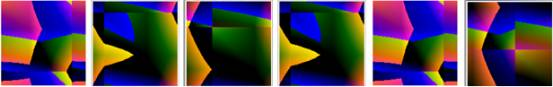Figure 5: Animated Expression Sequence

Expression:

`Distort(Distort(ColorGradient(), Average(0.8206732, Abs(Distort(Distort(ColorGradient(), Average(0.8206732, Abs(SawPulse()))), 0.8206732)))), 0.8206732)`

Second, a genetic morph kind of linear interpolation that is made possible by the genetic algorithms from which the behaviors originate. Originally described by Sims, this transition between two behaviors is accomplished by walking the expression trees in parallel from the roots. If the behaviors have a common ancestor, much of the structure will be similar between expressions. The interpolated expression retains however much of the structure is identical between them, then linearly interpolates between the nodes that are different. The interpolation parameter is time varying to produce a smooth transition between images.

The results that Sims presents from this kind of animation are visually astounding. The transition affects a morph between images in a manner that is completely alien to our common experience with simple distortion or blending because the interpolants are deeply nested in the expressions. This kind of transition is unique to behaviors created by genetic algorithms.

The genetic morph has two parts. A morphing expression is created, then animated by repeated interpolation of a blending parameter. The morphing expression is created by walking the begin and end expressions, creating a clone. Whenever part of the parse tree differs between them, a special expression is introduced: a MorphInterpolator.

 `// Resulting expression is a clone of a and b that interpolates``// between them.``Expression morph(Expression a, Expression b):`` ``  // The algorithm recurses in cases where the expressions are ``  // both calls and operators are the same and there are the same``  // number of arguments to each.  Detect this case.``  if (a instanceof ELCall) and (b instanceof ELCall)``     and (a.operator.equals(b.operator)) and ``    (a.numArgs == b.numArgs) then`` ``     return new Call(a.operator, ``                     morph(a.arg, b.arg), ``                     morph(a.arg, b.arg), ...)``  else``     // These are not the same call expression...``     // If these are the same expression, return that expression, ``     // otherwise return an interpolator.  Note that either way,``     // the recursion stops here.`` ``     if a.sameExpression(b) then``        return a.clone()``     else``        return new MorphInterpolator(a.clone(), b.clone())`

Figure 6:Genetic Morph Algorithm (Pseudo code)

The following is a morph animation sequence produced using Evolver.  Note how the structure of the image changes over time… this is not simple linear interpolation of pixels, and it does not require any of the sophisticated image search and registration capabilities of traditional image warping algorithms.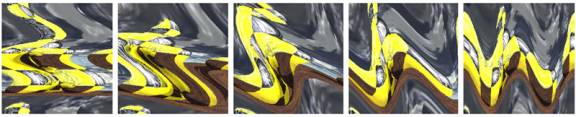Figure 7: Morph Animation Sequence (0%, 25%, 50%, 75%, 100%)

Expression A: ```Distort(RayTraceImage(), Cosine(ArcTangent(VGradient())))```

Expression B: ```Distort(RayTraceImage(), Cosine(HGradient()))```

Resulting Morph Expression:

```Distort(RayTraceImage(), Cosine(MorphInterpolator(ArcTangent(VGradient()), HGradient())))```

# V.        User Interface

## Evolver Window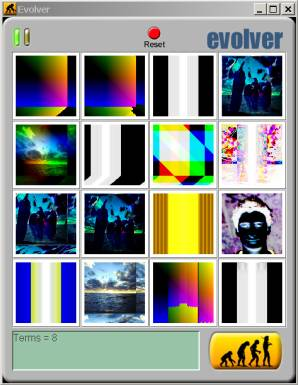Figure 8: Evolver Window

The Evolver window, shown above, is the user interface for creating and breeding generations of behaviors.  It consists of a status indicator light, a series of behaviors (a “generation”), some notational text, a reset button, and an evolve button.

The behaviors are arranged in a grid.  Each one capable of drag-drop, so they may be easily dragged into other windows and behaviors can be dropped onto the Evolver window.  The program loads with a generation of random behaviors.  The user selects one or more behaviors by single-clicking on them, then presses the yellow-orange evolve button.  Evolver mates the selected expressions randomly, producing a new generation.

At any point, the user can press the reset button to generate another random generation.  The status light blinks to indicate that Evolver is ready.  When it is not blinking, Evolver is busy computing a new generation.

The green text output box provides information about the behavior the mouse is over.  This includes the number of terms in the expression, whether it is animated, and what the root expression is.

## Behavior Viewer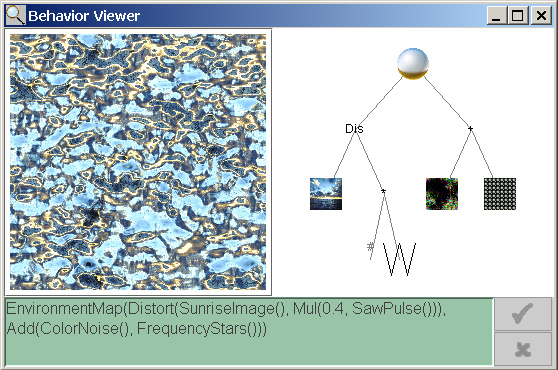Figure 9:Behavior Viewer

The Behavior Viewer displays a behavior and the expression for it.  The expression is shown as EL code in the green text box, as well as a parse tree in the graph on the upper right.  Note that since EL is a filter language, a parse tree is also a block diagram of a filter system (where the output is at the top and inputs are at the bottom).

Double clicking on the expression in the text box allows the user to edit the EL code manually.  Pressing the check button evaluates the new expression and the cross cancels the changes.  If the expression is animated, the behavior display will animate appropriately.  It is capable of 15-30fps for a 128x128 window.  In general, the image filter operations were not optimized.  I favored creation of many bug-free operators over a small set of fast ones.  To provide real-time animation capabilities, however, I did optimize the slower filters especially for the 128x128 resolution.  Blur, Distort, image sources, TimeSpinner and other complicated filters are approximately 10 times faster on 128x128 images than 128x127 images as a result.

To view a behavior, drag it from another window to the behavior rendering in the Behavior Viewer.  The behavior rendering may also be dragged to other windows.

The Behavior Viewer is useful for saving behaviors (just copy the text expression from the window) and loading them.  It displays behaviors animated and larger than the main Evolver window, assisting with fitness evaluation.  The parse graph is a powerful tool for quickly evaluating which behaviors will create suitable morphs.

## Morph Viewer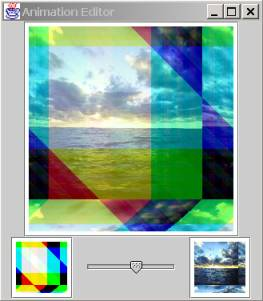Figure 10:Morph Viewer

The Morph Viewer allows previewing of a Genetic Morph between two behaviors, which is convenient when creating animation sequences from individual behaviors.

## Animation Editor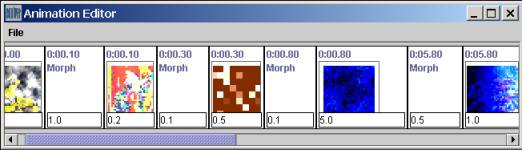Figure 11: Animation Editor

The animation editor allows graphical editing of an animation sequence.  Individual EL expressions can be dropped onto the thumbnail frames.  The resulting animation will consist of the expressions and morphs between them.  The user can type in a duration for each morph and for how long an expression should be displayed.  The width of a frame indicates the duration.  The number in the upper left is time code, useful for syncing animation to audio. Animation sequences can be saved out to human readable files that are playable by an external Evolver animation player.  These animation sequences are entirely procedural so they require very little storage space.

## Genetic Console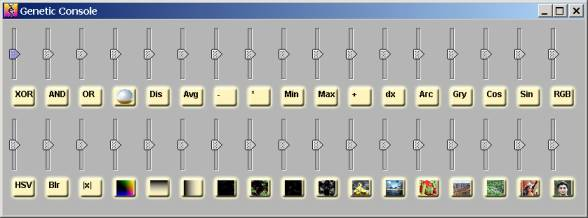Figure 12: Genetic Console

The genetic console is the graphic interface by which the user can influence the probability of any operator appearing.  Pushing the glowing buttons toggles whether a given operator will appear.  The sliders control the relative probability of any expression appearing, where up = maximum probability.  The file defaults.gp is used to initialize these values.  The user may also edit the text file directly before the program is loaded.  defaults.gp allows control over the mutation probabilities as well.  No GUI is provided for editing the mutation probabilities.

The list of operators is created using the Java reflection and dynamic class loading APIs.  Any .class file implementing the ELOperator interface is automatically loaded and added to the genetic parameters window.

# VI.       Source code

Evolver is implemented in Java and has been tested on Windows 2000 and Mac OS X (drag-drop does not work under OS X).  It should work on any compliant Java 1.3 platform.  The source is fully commented and documented for use with `javadoc`.

Because this project includes full implementations of a programming language (EL), animation editor, image filter package, genetic optimizer, and sophisticated GUI, there is a very large amount of source code—approximately 7500 lines of Java code.

The Evolver source code has been released for general use and is available on the web under a very open software license.  The imaging library and many of the GUI components can be used independently of the rest of the code, increasing their re-use potential.  The genetic algorithm implementation is fairly wedded to signal processing and is not well suited for use in other domains.  There are no known bugs in the code base.

Evolver is thread safe. On a multi-processor machine, it is possible to enable multi-threaded evaluation of a single Evolver expression to improve performance. Experiments showed that spawning a new thread for every sub-expression of a call did not improve performance on a dual processor Intel PII. However, multithreading evaluation of ELMorphInterpolators did reduce evaluation time by 36%. These tests were performed on the morph expression:

 `MorphInterpolator(``  Derivative(EnvironmentMap(Grayscale(Abs(Distort(LowColorNoise(),``             Abs(ToHSV(0.38841355))))),``             Sub(Min(ToHSV({0.15902214, -0.31222168, -0.04161206}), ``             LowNoise()), 0.26385573))),``  Blur(ToHSV(ToRGB(Add({0.03658315, -0.034329902, 0.5085707}, ``             ToRGB(Distort(Cosine(Derivative(ArcTangent(LowNoise()))), ``             ToRGB(Add({0.03658315, -0.034329902, 0.5085707}, ``             ColorGradient())))))))))`

Figure 13: Performance Test Expression

The single threaded time to evaluate this expression for a resolution of 128x128 pixels was 500ms. MorphInterpolator multi-threading reduced the time cost to 320ms. Adding call multi-threading increased the time to 650ms (these numbers are averages over multiple runs and have an error range of +/- 15ms).

The fields `Evolver.multiThreadEvaluation` and `Evolver.multiThreadInterpolation` control the multi-threading behavior of the program.

## Implementation Notes

It is very difficult to debug genetic algorithms because everything is probabilistic. The very nature of the algorithm is that it runs for a long time and the result is unpredictable.

Because it handles so many images and animations, Evolver takes up a lot of memory.  This can cause thrashing of virtual memory.  To avoid this, some images are rendered at very low resolution and enlarged (e.g. the thumbnails in the animation editor).  SoftReferences—one of the Java weak pointer variations—are used to allow the garbage collector to reclaim some cache data structures and improve memory performance.  The images used in computing expressions are explicitly allocated and deallocated using  buffer pooled storage (see ELMatrix.java).  Evolver requires approximately 80MB of free memory to run.  I recommend increasing the JVM’s heap to 100MB and enabling incremental garbage collection to improve performance (java -Xincgc -Xmx100M Evolver).

Drag-drop uses expression parsing and unparsing to serialize expressions.  Because EL expressions support Java-style comments, additional information can be embedded in comments.

Many parts of the GUI are connected using a code-efficient reflection scheme.  The BooleanFieldAdapter and DoubleFieldAdapter classes provide generic Java Swing style data models that connect javax.swing.* components directly to the fields of a class, rather than requiring separate data models for each control or losing the GUI/data model abstraction.

Operators are dynamically loaded using Java’s class loader and the reflection API.  Any abstract class implementing ELOperator that is found in the Evolver directory is automatically loaded.

# VII.     Results and Discussion

Evolver is capable of generating an incredibly large variety of images using its simple filter set.  The Image Gallery at the end of this section demonstrates this.  Although I was unable to exactly duplicate Sims’s images because he did not publish all of the input images or filters he used, I was able to generate images with the same kinds of effects.   I was also able to successfully reproduce the genetic morph.

My new technique of using time and frequency varying filters was successful.  It produces interesting animations that can be combined with the genetic morph.  Using both of these capabilities, I produced an animation that is synched to accompanying music (available on the web site).

## Image Gallery

These are actual, unretouched images produced by Evolver.  In each case the expression was generated by mating randomly generated expressions.  Some artifacts may appear from the JPEG compression or the page setting program’s scaling algorithm.  The expressions are given in text beneath each image; paste these into Evolver’s Behavior Viewer to recreate each image.  Controls from the Behavior Viewer are visible in the images.  These are different between the images because they are screen shots from different versions of the UI.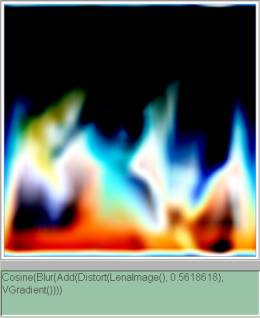Figure 14: Fire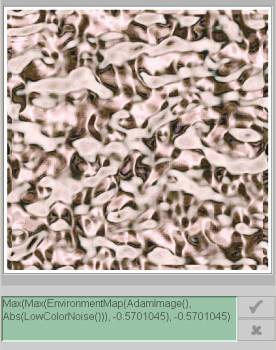Figure 15: Foil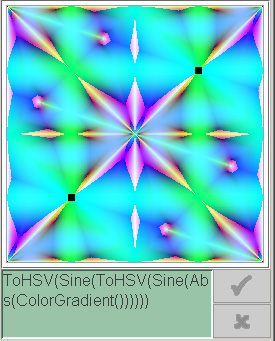Figure 16: Neon Quilt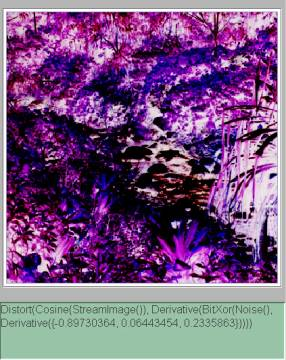Figure 17: Alien Landscape

Distort(Cosine(StreamImage()), Derivative(BitXor(Noise(), Derivative({-0.89730364, 0.06443453, 0.2335863}))))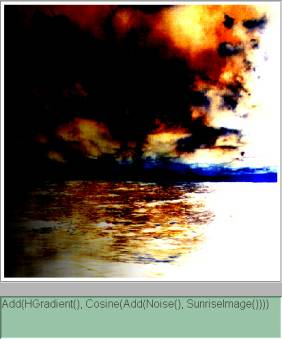Figure 18: Apocalypse Now

Some interesting animation expressions:

Raindrops on a sunny day

`EnvironmentMap(Distort(SunriseImage(), Mul(0.4, SawPulse())), Add(ColorNoise(), FrequencyStars()))`

Color shapes

`Distort(Distort(ColorGradient(), Average(0.8206732, Abs(Distort(Distort(ColorGradient(), Average(0.8206732, Abs(SawPulse()))), 0.8206732)))), 0.8206732)`

Undersea

`Add(Distort(Abs(Average(VGradient(), {0.21982141, 0.06335712, 0.8379867})), Add(Distort(Mul(0.22944131, ColorNoise()), Add(SawPulse(), 0.84141)), StreamImage())), Distort(FrequencyStars(), Mul(SawPulse(), 0.672345)))`

Disco

`Add(ColorGradient(), Sine(Sine(Blur(Min(0.71339244, Min(0.71339244, FrequencyBlocks()))))))`

Color cycler

`Abs(HSymmetry(BitOr(TimeSpinner(ColorGradient()), BitXor(0.25197303, DSymmetry(0.45049655)))))`

Psychedelic

`Abs(HSymmetry(TimeSpinner(Min(LowColorNoise(), Sine(Sub(SunriseImage(), LenaImage()))))))`

Blue curves

`ToHSV(Distort(FrequencyBlocks(), Distort(HGradient(), FrequencyBlocks())))`

Worms

`Derivative(Sine(Average(Average(Noise(), SawPulse()), {0.53519505, -0.03742808, -0.5621228})))`

Untitled series

`Blur(Sub(SawPulse(), Min(BitXor(-0.14766409, SawPulse()), BitOr(ColorGradient(), TimeSpinner({-0.15466845, 0.23334767, 0.31008685})))))`
` `
` `
`Blur(Sub(ToHSV(Blur(Sub(SawPulse(), ColorNoise()))), Noise()))`
` `
` `
`HSymmetry(Sub(LenaImage(), DSymmetry(Sine(Noise()))))`
` `
` `
`HSymmetry(Sub(LenaImage(), TimeSpinner(DSymmetry(Sine(Noise())))))`
` `
` `
`HSymmetry(Sub(0.8103099, TimeSpinner(ColorGradient())))`
` `
`Max(Blur(EnvironmentMap(HGradient(), Distort(DSymmetry(TimeSpinner(HGradient())), AdamImage()))), ColorGradient())`
`EnvironmentMap(Mul(0.80912614, Derivative(LowColorNoise())), Max(Mul(SawPulse(), AdamImage()), HGradient()))`
` `
` `
`EnvironmentMap(SunriseImage(), Max(Mul(SawPulse(), AdamImage()), HGradient()))`